Coming to Statistica
in 2022/2023

Zoom tutorials
using Explaineverything app
We a record sessions for you to listen to later

CALL STATISTICA NOW

.

E-mail     anne@statistica.com.au

# Place         Willetton, Western Australia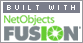# Mathematics & Statistic Tutor Perth - SPSS Help

Here are some simple logarithm questions where you are using the rules of logarithm.  Check on the rules here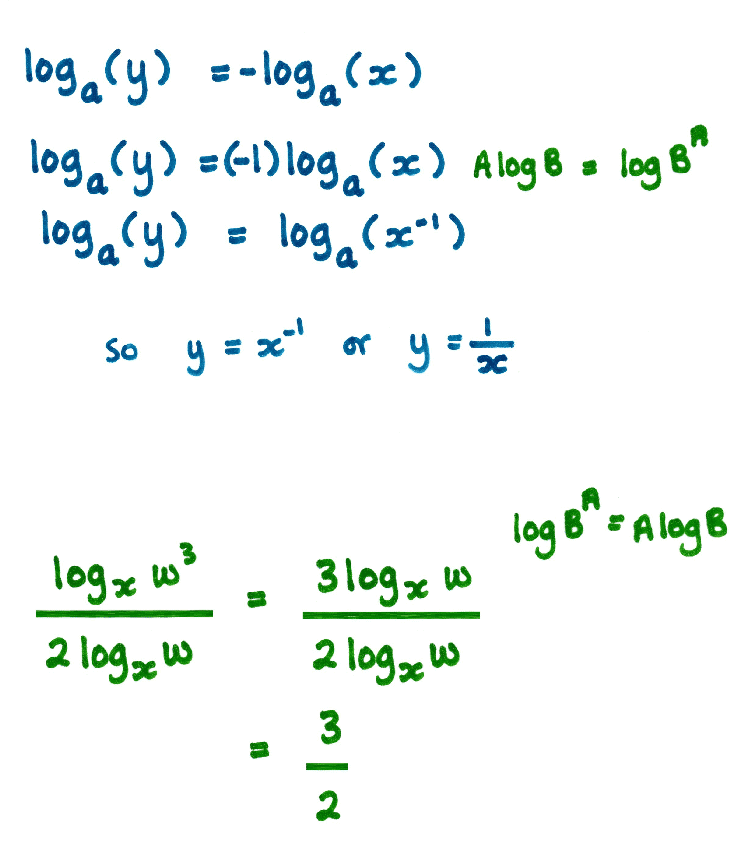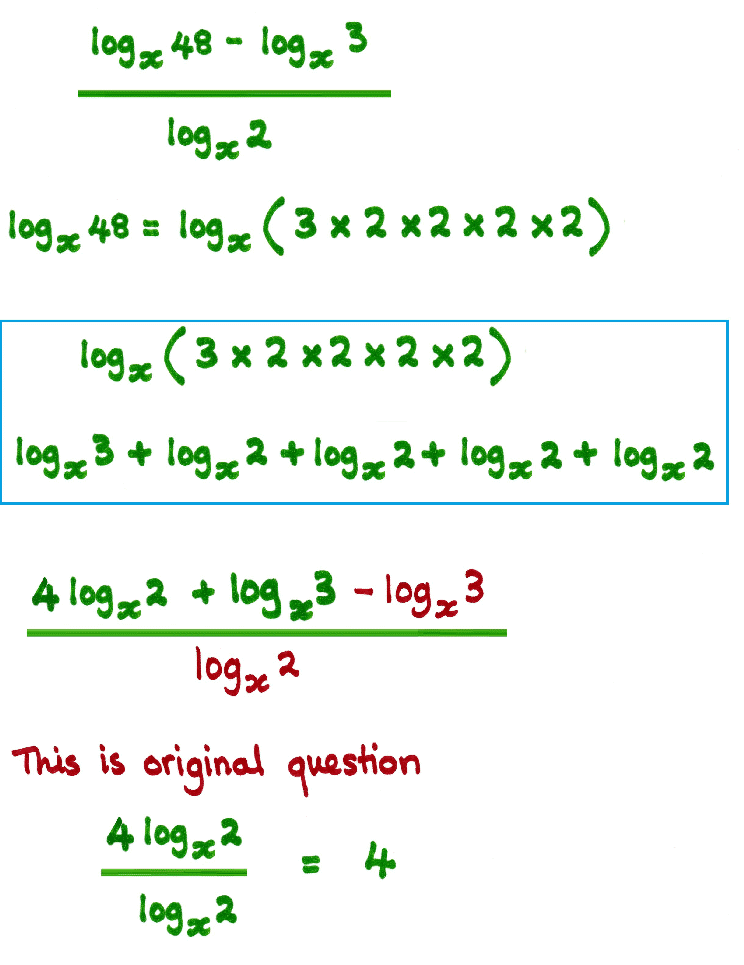Here is logarithm question where you are using the rules of logarithm to obtain a quadratic equation.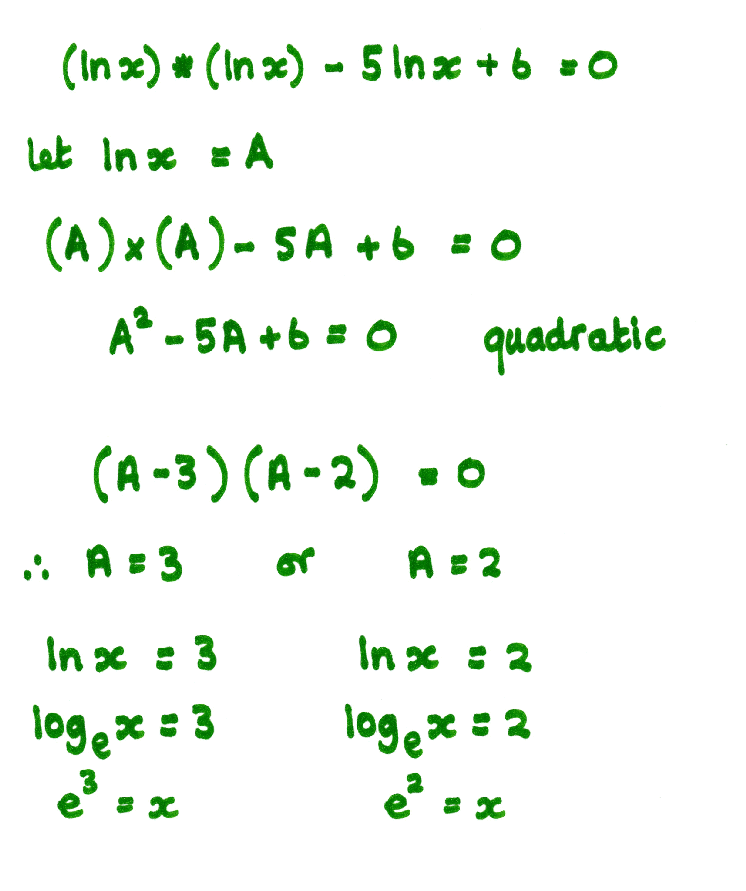Here are some more logarithm questions where you are changing Power Form to Logarithm Form and vice versa.  There are some videos to help, click the Frog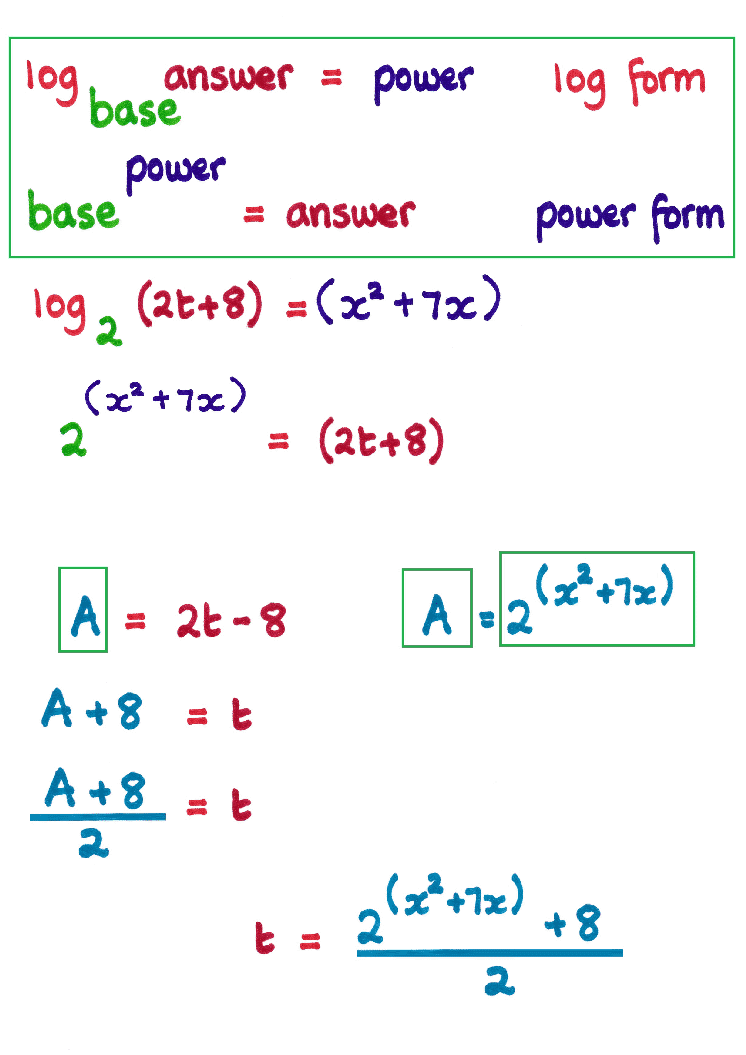[Home] [Parents] [Contact us] [Services] [Mathematics] [Statistics] [School]